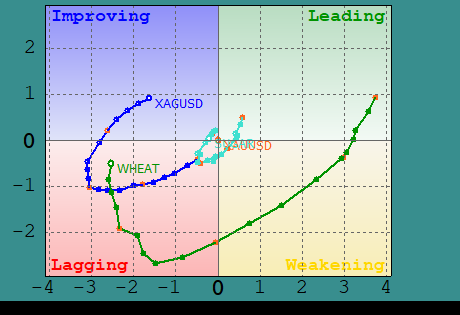### Stock Portfolio Organizer

The ultimate porfolio management solution.

Shares, Margin, CFD's, Futures and Forex
EOD and Realtime
Dividends and Trust Distributions
And Much More ....

#1 Selling Amibroker Plugin featuring:

Neural Networks
And Much More ....
Find Out More Here

### Rotasi Saham / Stock Rotation XY-Chart for Amibroker (AFL) newbie123 5 months ago Amibroker (AFL)

Rating:
Tags:
amibroker

Rotasi Saham digunakan untuk memetakan saham yang outperform / underperfom dibandingkan index acuan, ke dalam 4 kuadran XY chart.

- Kanan bawah = Weakening
- Kiri bawah = Lagging
- Kiri atas = Improving

Ini adalah versi kode paling dasar yang dibutuhkan.
Silakan menambahkan warna dan tulisan untuk masing2 kuadran sesuai keinginan kalian.

(translation)
Stock rotation is used to map stocks that outperform / underperform compared to the reference index, into 4 quadrants of the XY chart.

– Bottom right = Weakening
– Bottom left = Lagging
– Top left = Improving

This is the most basic version of the code needed.

### Indicator / Formula

```_SECTION_BEGIN( "XY-Rotation Chart" );

_N( base = ParamStr( "Base", "IHSG" ) );
_N( list = ParamStr( "Symbols", "IDXBASIC,IDXCYCLIC,IDXENERGY,IDXFINANCE,IDXHEALTH,IDXINDUST,IDXINFRA,IDXNONCYC,IDXPROPERT,IDXTECHNO,IDXTRANS" ) );
tbar = Param( "Trailing Bar", 12, 1, 100, 1 );

EnableTextOutput( False );
GfxSetOverlayMode( 2 );

pxl = Status( "pxchartleft" );
pxr = Status( "pxchartright" );
pxt = Status( "pxcharttop" );
pxb = Status( "pxchartbottom" );
pxw = ( pxr - pxl ) / 2;
pxh = ( pxb - pxt ) / 2;
xm = pxl + pxw;
ym = pxt + pxh;

GfxMoveTo( pxl, ym );
GfxLineTo( pxr, ym );
GfxMoveTo( xm, pxt );
GfxLineTo( xm, pxb );

function getrs ( sc, t )
{
bc = Foreign( base, "C" );
sbr = sc / bc;

rs1 = MA( sbr, 12 );
rs2 = MA( sbr, 26 );
rs = 100 * ( ( rs1 - rs2 ) / rs2 + 1 );

rm1 = MA( rs, 1 );
rm2 = MA( rs, 9 );
rm = 100 * ( ( rm1 - rm2 ) / rm2 + 1 );

return IIf( t, rs , rm ) - 100;
}

function drawpos ( x, y, sym, text )
{
rsl = VarGet( "rsl" );
rsh = VarGet( "rsh" );
rml = VarGet( "rml" );
rmh = VarGet( "rmh" );

xx = pxl + pxw + x * ( pxw / ( Max( rsh, -rsl ) * 1.10 ) );
yy = pxb - pxh - y * ( pxh / ( Max( rmh, -rml ) * 1.10 ) );

xp = Nz( VarGet( "xp" + sym ), xx );
yp = Nz( VarGet( "yp" + sym ), yy );

VarSet( "xp" + sym, xx );
VarSet( "yp" + sym, yy );

GfxMoveTo( xp, yp );
GfxLineTo( xx, yy );

GfxCircle( xx, yy, IIf( text == "", 2, 4 ) );

if ( text != "" ) GfxTextOut( sym, xx + 6 , yy - 3 );
}

for ( i = 0; ( sym = StrExtract( list, i ) ) != ""; i++ )
{
SetForeign( sym );

rs = getrs( C, 1 );
rm = getrs( C, 0 );

for ( ii = BarCount - tbar; ii < BarCount; ii++ )
{
rs_ = rs[ ii ];
rm_ = rm[ ii ];

rsh = Nz( VarGet( "rsh" ), rs_ );
if ( rs_ >= rsh )
VarSet( "rsh", rs_ );

rsl = Nz( VarGet( "rsl" ), rs_ );
if ( rs_ <= rsl )
VarSet( "rsl", rs_ );

rmh = Nz( VarGet( "rmh" ), rm_ );
if ( rm_ >= rmh )
VarSet( "rmh", rm_ );

rml = Nz( VarGet( "rml" ), rm_ );
if ( rm_ <= rml )
VarSet( "rml", rm_ );
}

RestorePriceArrays( True );
}

for ( i = 0; ( sym = StrExtract( list, i ) ) != ""; i++ )
{
SetForeign( sym );

rs = getrs( C, 1 );
rm = getrs( C, 0 );

for ( ii = BarCount - tbar; ii < BarCount; ii++ )
{
drawpos( rs[ ii ], rm[ ii ], sym, WriteIf( ii == BarCount - 1, sym, "" ) );
}

RestorePriceArrays( True );
}

_SECTION_END();```

1.

hi
chart empty , only 4 quadrants showed .
thanks

2.

in line 3, change the name of the index/stock you want to use as a reference/base

in line 4, change the name of the stock you want to display on the chart

3.

ok , thanx for replay

4.

to user :
to more understand how this afl work.

5.

6.

Thank you very much…

You inspired me and helped me improve the code. As a result, I was able to achieve the following result…

Thanks again…7.

Very nice TigerXXX. Good job and happy for you.

8.

9.

Hi TigerXXX,

10.

Hi TigerXXX,

12.

Thank you for sharing. Its quite helpful

13.

Thanks for your sharing, exactly what I was looking for. respect your contribution

14.

Hi TigerXXX,
Thank you very much in advance!

15.

thank bro for share

16.

17.

18.

Di ami sy tampilan nya blank ya ?

19.

Halo gan, boleh share contact telegram atau WA? mau minta tolong ada project. Salam.

20.

bang kok g ada gambarnya. gimana cara gunainnya

21.

Hi,
rs1 = MA;
rs2 = MA;
rs = 100 * ( ( rs1 – rs2 ) / rs2 + 1 );

rm1 = MA; rm2 = MA;

apakah angka pada parameter MA merupakan defaultnya atau bisa di set up lagi ? Tks.

22.

Hi,
rs1 = MA;
rs2 = MA;
rs = 100 * ( ( rs1 – rs2 ) / rs2 + 1 );

rm1 = MA; rm2 = MA;

apakah angka pada parameter MA merupakan defaultnya atau bisa di set up lagi ? Tks.